﻿ DecimalRange Class Members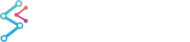Properties  Methods  Events

 SciChart.Data Assembly > SciChart.Data.Model Namespace : DecimalRange Class
DecimalRange Class Members

The following tables list the members exposed by DecimalRange.

Public Constructors
NameDescription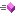Overloaded.
Top
Public Properties
NameDescription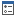Overridden. Gets the Diff (Max - Min) of this rangeGets whether this Range is defined (Inherited from SciChart.Data.Model.Range<decimal>)Overridden. Gets whether the range is Zero, where Max equals MinGets or sets the Max value of this range (Inherited from SciChart.Data.Model.Range<decimal>)Gets or sets the Min value of this range (Inherited from SciChart.Data.Model.Range<decimal>)
Top
Public Methods
NameDescriptionOverridden. Converts this range to a DoubleRange, which are used internally for calculationsOverloaded. Clips the current IRange to a maxmimum range with RangeClipMode.MinMax mode (Inherited from SciChart.Data.Model.Range<decimal>)Overridden. Creates a new object that is a copy of the current instance.Overloaded. Determines whether the specified object is equal to the current object. (Inherited from SciChart.Data.Model.Range<decimal>)Serves as the default hash function. (Inherited from SciChart.Data.Model.Range<decimal>)Overridden. Grows the current IRange<T> by the min and max fraction, returning this instance after modificationReturns True if the value is within the Min and Max of the Range (Inherited from SciChart.Data.Model.Range<decimal>)Overloaded. Overridden. Sets the Min, Max values on the IRange<T>, returning this instance after modificationSets the Min, Max values on the IRange<T>, returning this instance after modification (Inherited from SciChart.Data.Model.Range<decimal>)Returns a string that represents the current object. (Inherited from SciChart.Data.Model.Range<decimal>)Overloaded. Performs the Union of two IRange instances, returning a new IRange (Inherited from SciChart.Data.Model.Range<decimal>)
Top
Protected Methods
NameDescription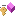Overloaded. Raises the System.ComponentModel.INotifyPropertyChanged.PropertyChanged event (Inherited from SciChart.Data.Model.BindableObject)Internal implementation: Sets the Min, Max values of the Range<T> (Inherited from SciChart.Data.Model.Range<decimal>)
Top
Extension Methods
NameDescriptionNotifies that finalizer has been invokedGrows current SciChart.Data.Model.IRange instance by minFraction, maxFraction in log scaleReturns a new SciChart.Data.Model.DateRange that corresponds to the provided range.Overloaded. Converts an System.IComparable array to double arrayYields a single item, converting it to System.Collections.IEnumerable.Returns a new SciChart.Data.Model.DateRange that corresponds to the provided range.
Top
Public Events
NameDescription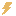Occurs when a property value changes. (Inherited from SciChart.Data.Model.BindableObject)
Top